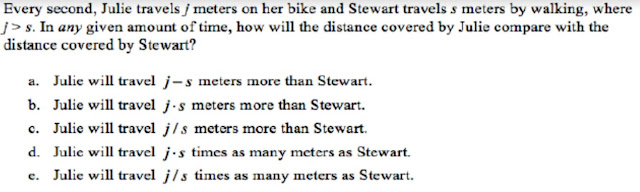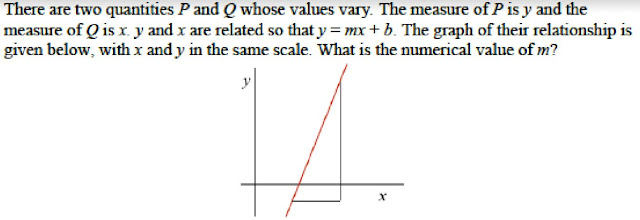## Thursday, November 1, 2018

### The Derivative is not the Slope of the Tangent Line

The title of this article is not intended to imply that one cannot use the derivative to find the slope of a tangent line. My point is that we cannot and should not expect students to base their understanding of the derivative on the slope of the tangent.

When I teach either the first or second semester of calculus, I always begin with a short problem set to assess student understanding of a few key ideas. One of the first questions I pose is to give the students a simple cubic polynomial, say x3+ 6x, and ask for both the average rate of change over a given interval, say [0,2], and the instantaneous rate of change at a particular value, say x = 1. Invariably, almost everyone, even at the start of Calculus I, can calculate the instantaneous rate of change. Almost no one gives me the correct average rate of change.

The difficulty is that finding the instantaneous rate is formulaic. If students remember nothing else from calculus, they know that differentiation turns x3+ 6x into 3x+ 6. Asking for the average rate of change requires that they know what this means. I am certain that my students all saw average rates of change in their precalculus courses. They probably saw it again when they were introduced to the derivative in high school calculus. But in a calculus class, it is merely a step in the development of the derivative, a case of what the teacher talks about but not what they need to know for the exam.

The belief that average rates of change are not significant is reinforced when, as in Stewart’s calculus, the derivative is introduced as the slope of the tangent line. The problem is that slope is a problematic concept for many students. Identifying the derivative with the slope of a tangent line suggests a geometric understanding of derivatives. But too often it does no such thing, instead short-circuiting student development of an understanding of the derivative as describing the multiplicative relationship between changes in two linked variables.

The problematic nature of slope and rates of change was nicely documented in a paper by Cameron Byerley and Pat Thompson that appeared last year in the Journal of Mathematical Behavior. In the summers of 2013 and 2014, they administered a diagnostic instrument requiring written responses to 251 high school mathematics teachers.

The following is an example of the kinds of questions that were asked. Part B was asked on a separate page with the answer entered by pen so that teachers could not go back to change the answer to Part A after seeing Part B.

Part A. Mrs. Samber taught an introductory lesson on slope. In the lesson she divided 8.2 by 2.7 to calculate the slope of a line, getting 3.04. Convey to Mrs. Samber’s students what 3.04 means.

Part B. A student explained the meaning of 3.04 by saying, “It means that every time x changes by 1, y changes by 3.04.” Mrs. Samber asked, “What would 3.04 mean if x changes by something other than 1?” What would be a good answer to Mrs. Samber’s question?

The point that Byerley and Thompson were getting at was whether teachers recognized 3.04 as a multiplicative factor connecting the change in x to the change in y. Earlier interviews had revealed that many teachers have a “chunky” understanding of slope, that a slope of ¾ means that if you go 4 units to the right and 3 units up, you will return to the line. One sign of a chunky understanding is an inability to find the increase in y if x changes by something other than 4. Another is the belief that a slope of  –5/6 is different from a slope of 5/–6, indicating that the teacher understands a slope of a/b as meaning a sequence of actions rather than a single number.

A chunky explanation of Part A, similar to the student’s response described in Part B, was given by 78% of the teachers. Part B was included to give them a chance to expand to a multiplicative explanation. Only 8% of the teachers who gave a chunky answer to Part A provided a multiplicative response to Part B.

Further teacher difficulties with the concept of slope and rate of change are illustrated in the following two problems (Figures 1 and 2).Figure 1. Item Called Relative Rates. © 2014 Arizona Board of Regents. Used with permission.
Most teachers interpreted the information in Figure 1 as describing a difference, with 54% answering a. Only 28% answered e.Figure 2. Item Called Slope from Blank Graph. © 2014 Arizona Board of Regents. Used with permission.
Only 21% of teachers were able to provide a reasonable approximation to the slope for the problem in Figure 2. Most were unable to give any numerical value.

Given teacher difficulties with the concept of slope, we should expect most of our students to enter calculus with an inadequate understanding of what it tells us about the relationship between the variables. While mathematicians hear “slope” and associate it with the multiplicative relationship between changes in the two variables, most of our students interpret it as nothing more than an arbitrary numerical description of the degree of “slantiness.”

Consequently, when we define the derivative as the slope of the tangent, we fail to convey the meaning that makes the derivative so useful. If we want students to understand this meaning, the derivative must be introduced in terms of a multiplicative relationship between changes in the variables. It must be grounded in a thorough understanding of what average rates of change tell us and what a constant rate of change actually implies.

Reference

Byerley, C. and Thompson, P. (2017). Secondary mathematics teachers’ meaning for measure, slope, and rate of change. Journal of Mathematical Behavior. 48:168–193.